# Getting Warmer: Smart Aiming With Heat-Seeking MissilesIn the previous tutorial we had a homing missile chasing after a single target. This tutorial will show you how to convert your homing missiles into heat-seeking missiles for multiple targets.

If you haven't read the first Homing Missile tutorial, you may download this .zip file, which contains the source code we'll be starting with on this tutorial.

## Final Result Preview

Let's take a look at the final result we will be working towards:

## Step 1: Modify the Cannon Graphic

The only Movie Clip in the Library we'll need to change is the Cannon, since we'll make it aim at the closest target before shooting. Remember that 0° of rotation means pointing at the right, so make the graphic accordingly.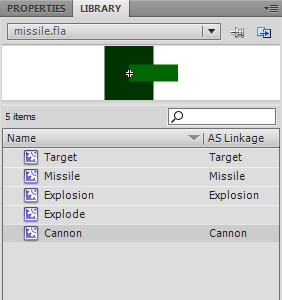## Step 2: Declare Distance Variables for the Cannon

I'm going to reuse the targetX and targetY variables to calculate the distance of the cannon from the target, so I'll declare them at the beginning of the class instead of inside the playGame function, as well as a new variable to store the calculated distance:

 1 2 private var missile:Missile = new Missile();  3 private var speed:int = 15;  4 private var cannon:Cannon = new Cannon();  5 private var missileOut:Boolean = false;  6 private var ease:int = 10;  7 private var target:Target = new Target();  8 private var floor:int = 385;  9 private var gravity:Number = 0.5;  10 private var targetVY:Number = 0;//Current vertical velocity of the target  11 private var distance:int;  12 private var targetX:int;  13 private var targetY:int; 

Now the targetX and targetY variables will be already declared for the playGame function:

 1 2 private function playGame(event:Event):void  3 {  4  if (missileOut)  5  {  6  if (missile.hitTestObject(target))  7  {  8  var explosion:Explosion = new Explosion();  9  addChild(explosion);  10  explosion.x = missile.x;  11  explosion.y = missile.y;  12  removeChild(missile);  13  missileOut = false;  14  }  15  else  16  {  17  targetX = target.x - missile.x;  18  targetY = target.y - missile.y;  19  var rotation:int = Math.atan2(targetY, targetX) * 180 / Math.PI;  20  if (Math.abs(rotation - missile.rotation) > 180)  21  {  22  if (rotation > 0 && missile.rotation < 0)  23  missile.rotation -= (360 - rotation + missile.rotation) / ease;  24  else if (missile.rotation > 0 && rotation < 0)  25  missile.rotation += (360 - rotation + missile.rotation) / ease;  26  }  27  else if (rotation < missile.rotation)  28  missile.rotation -= Math.abs(missile.rotation - rotation) / ease;  29  else  30  missile.rotation += Math.abs(rotation - missile.rotation) / ease;  31   32  var vx:Number = speed * (90 - Math.abs(missile.rotation)) / 90;  33  var vy:Number;  34  if (missile.rotation < 0)  35  vy = -speed + Math.abs(vx);  36  else  37  vy = speed - Math.abs(vx);  38   39  missile.x += vx;  40  missile.y += vy;  41  }  42  }  43  targetVY += gravity;  44  target.y += targetVY;  45  if (target.y > floor)  46  {  47  target.y = floor;  48  targetVY = -18;  49  }  50 } 

## Step 3: Make the Cannon Point Towards the Target

Previously in the playGame function we were only interested in knowing if the missile was out to take care of its rotation and motion. Now we need first to know if the missile hasn't been shot yet and update the cannon's rotation.

 1 2 private function playGame(event:Event):void  3 {  4  if (!missileOut)  5  {  6  targetX = target.x - cannon.x;  7  targetY = target.y - cannon.y;  8  cannon.rotation = Math.atan2(targetY, targetX) * 180 / Math.PI;  9  }  10  else  11  {  12  if (missile.hitTestObject(target))  13  {  14  var explosion:Explosion = new Explosion();  15  addChild(explosion);  16  explosion.x = missile.x;  17  explosion.y = missile.y;  18  removeChild(missile);  19  missileOut = false;  20  }  21  else  22  {  23  targetX = target.x - missile.x;  24  targetY = target.y - missile.y;  25  var rotation:int = Math.atan2(targetY, targetX) * 180 / Math.PI;  26  if (Math.abs(rotation - missile.rotation) > 180)  27  {  28  if (rotation > 0 && missile.rotation < 0)  29  missile.rotation -= (360 - rotation + missile.rotation) / ease;  30  else if (missile.rotation > 0 && rotation < 0)  31  missile.rotation += (360 - rotation + missile.rotation) / ease;  32  }  33  else if (rotation < missile.rotation)  34  missile.rotation -= Math.abs(missile.rotation - rotation) / ease;  35  else  36  missile.rotation += Math.abs(rotation - missile.rotation) / ease;  37   38  var vx:Number = speed * (90 - Math.abs(missile.rotation)) / 90;  39  var vy:Number;  40  if (missile.rotation < 0)  41  vy = -speed + Math.abs(vx);  42  else  43  vy = speed - Math.abs(vx);  44   45  missile.x += vx;  46  missile.y += vy;  47  }  48  }  49  targetVY += gravity;  50  target.y += targetVY;  51  if (target.y > floor)  52  {  53  target.y = floor;  54  targetVY = -18;  55  }  56 } 

Now the cannon rotates in relation to the target's position.

## Step 4: Match the Missile's Rotation with the Cannon's.

The cannon is rotating, but the missile keeps being shot upwards. Replace the hard-coded rotation with the cannon's current location at the moment the missile is being shot.

 1 2 private function shoot(event:MouseEvent):void  3 {  4  if (!missileOut)  5  {  6  addChild(missile);  7  swapChildren(missile, cannon);//missile will come out from behind cannon  8  missileOut = true;  9  missile.x = cannon.x;  10  missile.y = cannon.y;  11  missile.rotation = cannon.rotation;  12  }  13 } 

Now the missile will look like it's actually coming out of the cannon.

## Step 5: More than One Target

Right now the homing missile is program to go after one target, but what if we have more targets? How will it decide which one to go after?

First, let's decide how many targets will there be, then we'll put each target in an Array. In this example I'm going to say there are 2 targets, and I'll give each target a random position on screen.

 1 2 private var target:Target;  3 private var floor:int = 385;  4 private var gravity:Number = 0.5;  5 private var targetVY:Number = 0;//Current vertical velocity of the target  6 private var distance:int;  7 private var targetX:int;  8 private var targetY:int;  9 private var numTargets:int = 2;  10 private var targets:Array = [];  11 12 public function Main()  13 {  14  addChild(cannon);  15  cannon.x = 50;  16  cannon.y = 380;  17  addEventListener(Event.ENTER_FRAME, playGame);  18  stage.addEventListener(MouseEvent.CLICK, shoot);  19  for (var i:int = 0; i < numTargets; i++)  20  {  21  target = new Target();  22  addChild(target);  23  target.x = Math.random() * 600;  24  target.y = Math.random() * 400;  25  targets.push(target);  26  }  27 } 

Now we have more than one target on screen.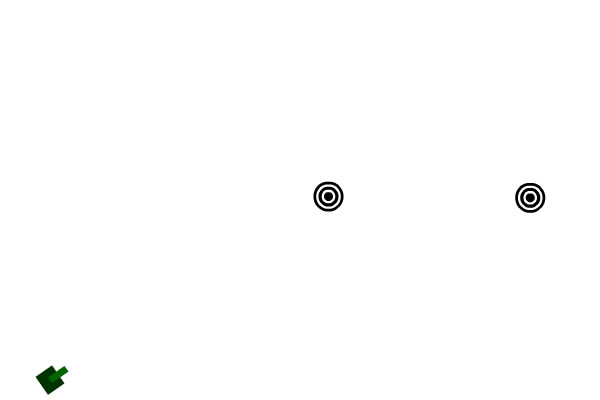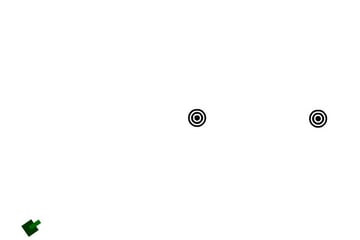The missile is still only acknowledging the existence of one target. We'll fix that next.

## Step 6: Seek the Closest Target

We have the missile seeking the target variable, so let's check the targets Array and see which one is closer. The target variable will be referencing the closest at the beginning of the playGame function.

 1 2 private function playGame(event:Event):void  3 {  4  for (var i:int = 0; i < targets.length; i++)  5  {  6  targetX = targets[i].x - missile.x;  7  targetY = targets[i].y - missile.y;  8  var dist:int = Math.sqrt(targetX * targetX + targetY * targetY);//the distance from one point to another in a 2D space.  9  if (i == 0 || dist < distance)  10  {  11  distance = dist;  12  target = targets[i];  13  }  14  } 

At this point the closest target is the only one moving, but the missile is acknowledging the existence of both:

## Step 7: Fix the Cannon's Aim

You may have noticed that while the missile does seek the expected target, the cannon's stuck pointing at the same target, regardless if it's closer or farther than the other one. The distance is set in relation to the missile's position, so if there's no missile on stage we need to update its position to match the cannon's so that it always knows which one is closer.

 1 2 private function playGame(event:Event):void  3 {  4  for (var i:int = 0; i < targets.length; i++)  5  {  6  targetX = targets[i].x - missile.x;  7  targetY = targets[i].y - missile.y;  8  var dist:int = Math.sqrt(targetX * targetX + targetY * targetY);  9  if (i == 0 || dist < distance)  10  {  11  distance = dist;  12  target = targets[i];  13  }  14  }  15  if (!missileOut)  16  {  17  missile.x = cannon.x;  18  missile.y = cannon.y;  19  targetX = target.x - cannon.x;  20  targetY = target.y - cannon.y;  21  cannon.rotation = Math.atan2(targetY, targetX) * 180 / Math.PI;  22  } 

Now the cannon will always aim at the closest target.

## Step 8: Move the Cannon

Before the missile is shot, the cannon is already pointing to the closest target, and will change direction if moved closer to the other target. Let's add a couple of lines to position the cannon with the mouse cursor.

 1 2 private function playGame(event:Event):void  3 {  4  cannon.x = mouseX;  5  cannon.y = mouseY; 

Now you can move the cannon freely.

## Step 9: Target Hit, Target Destroyed

To make things more dynamic here, I'm going to relocate a target after being hit by a missile, or replace it by a new one, and leave an Explosion instance in its place.

 1 2 if (missile.hitTestObject(target))  3 {  4  var explosion:Explosion = new Explosion();  5  addChild(explosion);  6  explosion.x = missile.x;  7  explosion.y = missile.y;  8  removeChild(missile);  9  missileOut = false;  10  explosion= new Explosion();  11  addChild(explosion);  12  explosion.x = target.x;  13  explosion.y = target.y;  14  explosion.scaleX = explosion.scaleY = 1.5;  15  target.x = Math.random() * 600;  16 } 

This is what you'll get:

## Step 10: Multiple Missiles

We made multiple targets, so now we can make multiple missiles the same way. The difference here is that all missiles must keep moving at all times until they hit, and we're actually going to remove those which have already exploded, so we need to modify a few lines of our code for this to work. First, we'll need an Array for the missiles.

 1 2 private var missiles:Array = []; 

Then, we need to make sure all the missiles behave properly:

 1 2 private function playGame(event:Event):void  3 {  4  cannon.x = mouseX;  5  cannon.y = mouseY;  6  for (var i:int = 0; i < targets.length; i++)  7  {  8  targetX = targets[i].x - missile.x;  9  targetY = targets[i].y - missile.y;  10  var dist:int = Math.sqrt(targetX * targetX + targetY * targetY);  11  if (i == 0 || dist < distance)  12  {  13  distance = dist;  14  target = targets[i];  15  }  16  }  17  if (!missileOut)  18  {  19  missile.x = cannon.x;  20  missile.y = cannon.y;  21  targetX = target.x - cannon.x;  22  targetY = target.y - cannon.y;  23  cannon.rotation = Math.atan2(targetY, targetX) * 180 / Math.PI;  24  }  25  else  26  {  27  for (i = 0; i < missiles.length; i++)//each missile must keep moving  28  {  29  missile = missiles[i];  30  if (missile.hitTestObject(target))  31  {  32  var explosion:Explosion = new Explosion();  33  addChild(explosion);  34  explosion.x = missile.x;  35  explosion.y = missile.y;  36  removeChild(missile);  37  missiles.splice(i, 1);//out of the Array  38  if (missiles.length < 1)//only if no missiles are out at all  39  missileOut = false;  40  explosion= new Explosion();  41  addChild(explosion);  42  explosion.x = target.x;  43  explosion.y = target.y;  44  explosion.scaleX = explosion.scaleY = 1.5;  45  target.x = Math.random() * 600;  46  }  47  else  48  {  49  targetX = target.x - missile.x;  50  targetY = target.y - missile.y;  51  var rotation:int = Math.atan2(targetY, targetX) * 180 / Math.PI;  52  if (Math.abs(rotation - missile.rotation) > 180)  53  {  54  if (rotation > 0 && missile.rotation < 0)  55  missile.rotation -= (360 - rotation + missile.rotation) / ease;  56  else if (missile.rotation > 0 && rotation < 0)  57  missile.rotation += (360 - rotation + missile.rotation) / ease;  58  }  59  else if (rotation < missile.rotation)  60  missile.rotation -= Math.abs(missile.rotation - rotation) / ease;  61  else  62  missile.rotation += Math.abs(rotation - missile.rotation) / ease;  63   64  var vx:Number = speed * (90 - Math.abs(missile.rotation)) / 90;  65  var vy:Number;  66  if (missile.rotation < 0)  67  vy = -speed + Math.abs(vx);  68  else  69  vy = speed - Math.abs(vx);  70   71  missile.x += vx;  72  missile.y += vy;  73  }  74  }  75  }  76  targetVY += gravity;  77  target.y += targetVY;  78  if (target.y > floor)  79  {  80  target.y = floor;  81  targetVY = -18;  82  }  83 }  84 85 private function shoot(event:MouseEvent):void  86 {  87  missile = new Missile();  88  missiles.push(missile);//into the Array  89  addChild(missile);  90  swapChildren(missile, cannon);//missile will come out from behind cannon  91  missileOut = true;  92  missile.x = cannon.x;  93  missile.y = cannon.y;  94  missile.rotation = cannon.rotation;  95 } 

Now when a target is destroyed, the missiles will seek the next target.

At this point all the missiles are chasing after the same target. In order to make each missile seek its own target, it would be better to make a separate class for the missiles in which you determine the closest target individually.

## Step 11: Make a Crosshair

At this point you've already understood the main idea of this tutorial, but let's face it, an enemy will not move depending only on its distance to you or your missiles. You can use another indicator, such as a crosshair. Make it a Movie Clip and export it to Actionscript.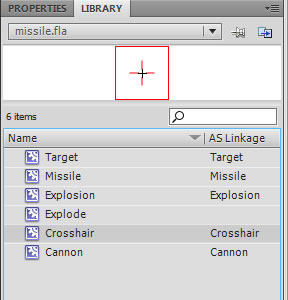## Step 12: Use the Crosshair

Now it will be obvious to anyone which target is being aimed at. Just add an instance of the Crosshair Movie Clip.

 1 2 private var crosshair:Crosshair = new Crosshair();  3 4 public function Main()  5 {  6  addChild(cannon);  7  cannon.x = 50;  8  cannon.y = 380;  9  addEventListener(Event.ENTER_FRAME, playGame);  10  stage.addEventListener(MouseEvent.CLICK, shoot);  11  for (var i:int = 0; i < numTargets; i++)  12  {  13  target = new Target();  14  addChild(target);  15  target.x = Math.random() * 600;  16  target.y = Math.random() * 400;  17  targets.push(target);  18  }  19  addChild(crosshair);  20 } 

Then place it on the target's position as the last instruction in the playGame function.

 1 2  targetVY += gravity;  3  target.y += targetVY;  4  if (target.y > floor)  5  {  6  target.y = floor;  7  targetVY = -18;  8  }  9  crosshair.x = target.x;  10  crosshair.y = target.y;  11 } 

You'll get a crosshair marking the closest target's position.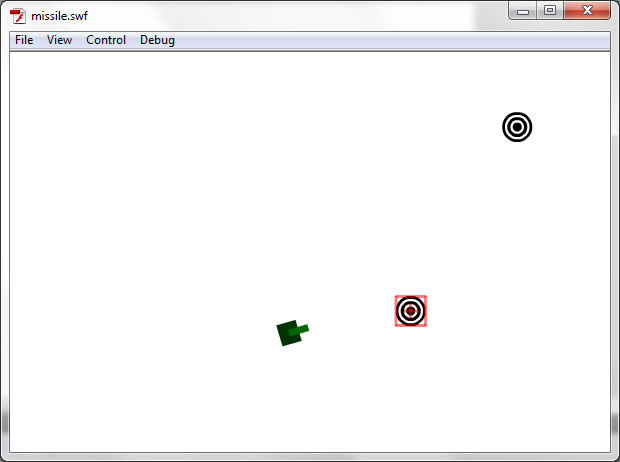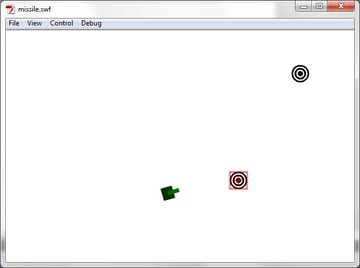## Step 13: Move All Targets

Remember what I said about the missiles? The same applies to the targets: They'll look better in their own class with a set of instructions of its own. This is just a quick example, but in your game I don't recommend coding all the objects in the Main class. The more complex your game is, the less stuff you'll code inside the Main class.

The targets are already in an Array, which is already being checked in a for loop, so I'll move the bouncing instructions inside the for loop, so that all the targets, no matter how many, will move the same at all times.

 1 2 private function playGame(event:Event):void  3 {  4  cannon.x = mouseX;  5  cannon.y = mouseY;  6  targetVY += gravity;  7  for (var i:int = 0; i < targets.length; i++)  8  {  9  targetX = targets[i].x - missile.x;  10  targetY = targets[i].y - missile.y;  11  var dist:int = Math.sqrt(targetX * targetX + targetY * targetY);  12  if (i == 0 || dist < distance)  13  {  14  distance = dist;  15  target = targets[i];  16  }  17  targets[i].y += targetVY;  18  if (targets[i].y > floor)  19  targets[i].y = floor;  20  }  21  if (target.y >= floor)  22  targetVY = -18;  23  if (!missileOut)  24  {  25  missile.x = cannon.x;  26  missile.y = cannon.y;  27  targetX = target.x - cannon.x;  28  targetY = target.y - cannon.y;  29  cannon.rotation = Math.atan2(targetY, targetX) * 180 / Math.PI;  30  }  31  else  32  {  33  for (i = 0; i < missiles.length; i++)  34  {  35  missile = missiles[i];  36  if (missile.hitTestObject(target))  37  {  38  var explosion:Explosion = new Explosion();  39  addChild(explosion);  40  explosion.x = missile.x;  41  explosion.y = missile.y;  42  removeChild(missile);  43  missiles.splice(i, 1);  44  if (missiles.length < 1)  45  missileOut = false;  46  explosion= new Explosion();  47  addChild(explosion);  48  explosion.x = target.x;  49  explosion.y = target.y;  50  explosion.scaleX = explosion.scaleY = 1.5;  51  target.x = Math.random() * 600;  52  }  53  else  54  {  55  targetX = target.x - missile.x;  56  targetY = target.y - missile.y;  57  var rotation:int = Math.atan2(targetY, targetX) * 180 / Math.PI;  58  if (Math.abs(rotation - missile.rotation) > 180)  59  {  60  if (rotation > 0 && missile.rotation < 0)  61  missile.rotation -= (360 - rotation + missile.rotation) / ease;  62  else if (missile.rotation > 0 && rotation < 0)  63  missile.rotation += (360 - rotation + missile.rotation) / ease;  64  }  65  else if (rotation < missile.rotation)  66  missile.rotation -= Math.abs(missile.rotation - rotation) / ease;  67  else  68  missile.rotation += Math.abs(rotation - missile.rotation) / ease;  69   70  var vx:Number = speed * (90 - Math.abs(missile.rotation)) / 90;  71  var vy:Number;  72  if (missile.rotation < 0)  73  vy = -speed + Math.abs(vx);  74  else  75  vy = speed - Math.abs(vx);  76   77  missile.x += vx;  78  missile.y += vy;  79  }  80  }  81  }  82  crosshair.x = target.x;  83  crosshair.y = target.y;  84 } 

Take a look:

## Conclusion

Homing missiles, heat-seeking missiles, both are a fun and useful weapon to have around in a shooting game or maybe some other type of app. This tutorial shows an example of its use and the algorithm to make it, but for best practices it is recommended that you have separate classes for the missiles and the targets, unless your app is as simple and short as this one.

I hope you've found this tutorial useful. Thanks for reading!